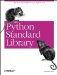# The code Module

The code module provides a number of functions that can be used to emulate the behavior of the standard interpreter's interactive mode.

The compile_command behaves like the built-in compile function, but does some additional tests to make sure you pass it a complete Python statement.

In Example 2-47, we're compiling a program line by line, executing the resulting code objects as soon as we manage to compile. The program looks like this:

```a = (
1,
2,
3
)
print a```

Note that the tuple assignment cannot be properly compiled until we've reached the second parenthesis.

Example 2-47. Using the code Module to Compile Statements

```File: code-example-1.py

import code
import string

#
SCRIPT = [
"a = (",
" 1,",
" 2,",
" 3 ",
")",
"print a"
]

script = ""

for line in SCRIPT:
script = script + line + "
"
co = code.compile_command(script, "", "exec")
if co:
# got a complete statement. execute it!
print "-"*40
print script,
print "-"*40
exec co
script = ""

----------------------------------------
a = (
1,
2,
3
)
----------------------------------------
----------------------------------------
print a
----------------------------------------
(1, 2, 3)```

The InteractiveConsole class implements an interactive console, much like the one you get when you fire up the Python interpreter in interactive mode.

The console can be either active (it calls a function to get the next line) or passive (you call the push method when you have new data). The default is to use the built-in raw_input function. Overload the method with the same name if you prefer to use another input function. Example 2-48 shows how to use the code module to emulate the interactive interpreter.

Example 2-48. Using the code Module to Emulate the Interactive Interpreter

```File: code-example-2.py

import code

console = code.InteractiveConsole()
console.interact()

Python 1.5.2
Copyright 1991-1995 Stichting Mathematisch Centrum, Amsterdam
(InteractiveConsole)
>>> a = (
... 1,
... 2,
... 3
... )
>>> print a
(1, 2, 3)```

The script in Example 2-49 defines a function called keyboard. It allows you to hand control over to the interactive interpreter at any point in your program.

Example 2-49. Using the code Module for Simple Debugging

```File: code-example-3.py

def keyboard(banner=None):
import code, sys

# use exception trick to pick up the current frame
try:
raise None
except:
frame = sys.exc_info().tb_frame.f_back

# evaluate commands in current namespace
namespace = frame.f_globals.copy()
namespace.update(frame.f_locals)

code.interact(banner=banner, local=namespace)

def func():
print "START"
a = 10
keyboard()
print "END"

func()

START
Python 1.5.2
Copyright 1991-1995 Stichting Mathematisch Centrum, Amsterdam
(InteractiveConsole)
>>> print a
10
>>> print keyboard

^Z
END```Python Standard Library (Nutshell Handbooks) with
ISBN: 0596000960
EAN: 2147483647
Year: 2000
Pages: 252
Authors: Fredrik Lundh